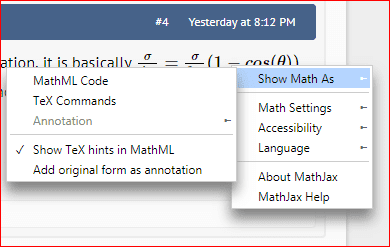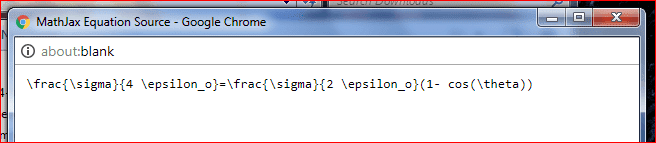# Electric field above a disk that equals half the max electric field

## Homework Statement

At what distance, a, along the axis of a uniformly charged disk of radius R is the axial electric field strength equal to one half its value at the surface of the disk at the center.

## Homework Equations

electric field of disk (attached)

## The Attempt at a Solution

First I derived the electric field of a disk

E=σ/2ε0 [1-(a/(x^2 +R^2)^(1/2))] (attached).

This problem wants us to look at where a<<R, Therefore the new electric field equation in that limiting case is:

E=σ/2ε0

If we want to know what "a" distance will half this electric field we:

(σ/4piε0) = σ/2ε0 [1-(a/(x^2 +R^2)^(1/2))] and solve for a?

I have attached my work, Attachments are labeled accordingly.

My final result for a-
a= (x^2 + R^2)^(1/2) / 2

[/B]

#### Attachments

Homework Helper
Gold Member
Your original formula should read ## E_z=(\frac{\sigma}{2 \epsilon_o})(1-\frac{z}{\sqrt{z^2+R^2}} ) ##. Otherwise you approach is correct, but you need to have ## z=a ## in both places. (Usually ## z ## is used for the on-axis direction).

•Your original formula should read ## E_z=(\frac{\sigma}{2 \epsilon_o})(1-\frac{z}{\sqrt{z^2+R^2}} ) ##. Otherwise you approach is correct, but you need to have ## z=a ## in both places. (Usually ## z ## is used for the on-axis direction).
Yes, I originally derived the disk of charge using other variable. but I see what you're saying.
Thanks a bunch charles!
You're awesome!!!!

•Homework Helper
Gold Member
In this problem, there is a long way to solve for ## a ##, but also a shortcut. If you look at the equation, it is basically ## \frac{\sigma}{4 \epsilon_o}=\frac{\sigma}{2 \epsilon_o}(1- cos(\theta)) ##. Clearly ## cos(\theta)=1/2 ## and that angle is one you should know. (In post 1 I see you have an incorrect ## pi ## in the denominator on the left side).

•SammyS
Staff Emeritus
Homework Helper
Gold Member

## Homework Statement

At what distance, a, along the axis of a uniformly charged disk of radius R is the axial electric field strength equal to one half its value at the surface of the disk at the center.

## Homework Equations

electric field of disk (attached)
Those attached pages a nearly impossible for me to read.
I know Charles was able to decipher these and the ones with your previous thread, but such difficult to read images are discouraged on these Forums.
Also, try to use BOLD fonts only for the template supplied titles.

## The Attempt at a Solution

First I derived the electric field of a disk

E=σ/2ε0 [1-(a/(x^2 +R^2)^(1/2))] (attached).[/B]
What is the x variable here?
There are icons for subscripts and superscripts in the blue banner line about text input box: X2 , X2 . Otherwise, a " _ " may work for subscript, much as you used " ^ " for superscript.
Also, a fraction such as σ/2ε0 literally means (σ/2)⋅(ε0) according to rules for order of operations. If you want to write such a fraction using 'inline' form put the denominator in parentheses. σ/(2ε0) or make it look really good by using LaTeX ##\displaystyle \ \frac{\sigma}{2\,\varepsilon_0} ##
This problem wants us to look at where a<<R, Therefore the new electric field equation in that limiting case is:

E=σ/(2 ε_0)

If we want to know what "a" distance will half this electric field we:

(σ/4piε0) = σ/2ε0 [1-(a/(x^2 +R^2)^(1/2))] and solve for a?
Where did that pi come from in the denominator on the left ?

I have attached my work, Attachments are labeled accordingly.

My final result for a-
a= (x^2 + R^2)^(1/2) / 2

[/B]
Again: What is ' x ' doing there ?

•Those attached pages a nearly impossible for me to read.
I know Charles was able to decipher these and the ones with your previous thread, but such difficult to read images are discouraged on these Forums.
Also, try to use BOLD fonts only for the template supplied titles.What is the x variable here?
There are icons for subscripts and superscripts in the blue banner line about text input box: X2 , X2 . Otherwise, a " _ " may work for subscript, much as you used " ^ " for superscript.
Also, a fraction such as σ/2ε0 literally means (σ/2)⋅(ε0) according to rules for order of operations. If you want to write such a fraction using 'inline' form put the denominator in parentheses. σ/(2ε0) or make it look really good by using LaTeX ##\displaystyle \ \frac{\sigma}{2\,\varepsilon_0} ##
Where did that pi come from in the denominator on the left ?
Again: What is ' x ' doing there ?
Okay! thanks for the input, I will either write my work out in a neater fashion or just learn to use the blue bar to write equations/work out.

Yes I used "x" instead of "a" (definitely confusing) sorry. I was working from a previously derived equation that used different variables, should have switched back.
To answer your question the x was meant to be the distance from the center of the disk (on axis).

After looking back at my work I believe the pi is incorrect (so used to writing 1/(4piε0)

As long as the work is neat and easy to follow are pictures accepted?

Thanks

Those attached pages a nearly impossible for me to read.
I know Charles was able to decipher these and the ones with your previous thread, but such difficult to read images are discouraged on these Forums.
Also, try to use BOLD fonts only for the template supplied titles.What is the x variable here?
There are icons for subscripts and superscripts in the blue banner line about text input box: X2 , X2 . Otherwise, a " _ " may work for subscript, much as you used " ^ " for superscript.
Also, a fraction such as σ/2ε0 literally means (σ/2)⋅(ε0) according to rules for order of operations. If you want to write such a fraction using 'inline' form put the denominator in parentheses. σ/(2ε0) or make it look really good by using LaTeX ##\displaystyle \ \frac{\sigma}{2\,\varepsilon_0} ##
Where did that pi come from in the denominator on the left ?
Again: What is ' x ' doing there ?
Could you explain how you do that LaTex thing with the division?

SammyS
Staff Emeritus
Homework Helper
Gold Member
•Homework Helper
Gold Member
@cookiemnstr510510 Although you might find "Latex" kind of slow when you are first using it, I also recommend you try to use it, if possible. For a while, you might find yourself needing to do a "google" to figure out how do do something in Latex, but with a little practice, you are likely to get pretty good with using it. ## \\ ## I myself had only used Latex for typing up one paper before joining the Physics Forums two and a half years ago, but I also got better at it with a little practice. It is somewhat slower than doing things by hand, but it's manageable.

•SammyS
Staff Emeritus
Homework Helper
Gold Member
Could you explain how you do that LaTex thing with the division?
I could have said more than that, but I was busy trying to juggle answering a couple of other threads at the time.

Some helpful points in using Latex .

Find a an expression that somebody has typeset in Latex. Right click on that expression. A window pops up as below. Click on "Show Math As" then choose Tex Commands.I did this for the first equation found in Post #4 of this thread, written by Charles and the following browser window popped up.See the Latex code which produced: ##\ \frac{\sigma}{4 \epsilon_o}=\frac{\sigma}{2 \epsilon_o}(1- cos(\theta)) \ ## in that post.
Notice that if you use the "Reply" feature in answering a thread, the Latex code will show in the message input box along with the rest of the Post, and the Latex code will include the # signs or $signs mentioned in the following. What is not displayed in that is that the code must either place a pair of pound signs, ## , before and a pair after the Latex to get an 'inline' expression, like in Post #4, else use a pair of dollar signs,$\$ , before and after. This will display your expression centered on its own line. There are other differences in the way these two options display the expressions, most notably the height of expressions, including fractions.

A few pointers about the Latex coding.
Many Latex commands and special features are preceded by the backslash character, \ . Notice the Greek characters \sigma, \epsilon, etc.
Fractions: Use ' \frac ' followed by two set of braces, { } { } . ( No division symbol for this form. ) In first set of { } , place the numerator, in the second set place the denominator.
For superscripts and/or exponents use the caret character ' ^ ' (No ' \ ' here ) followed by { } with the superscript expression to be inserted between the braces. Similarly, for subscripts, Use ' _ ' followed by the text of the subscript, of course surrounded by { }.
Note: For most of these Latex codes which use the brackets, if you are only placing a single character in the brackets, you don't need the brackets, but it's fine to always use them.​

Latex pretty much always ignores spaces. Well, you may need a space to separate pieces of Latex code, but Latex will not display any spaces you may be intending to display, unless you code the spaces. A \ followed by a space gives one space.
A \ followed by a comma, ' \, ' gives a small space. A ' \quad ' gives four normal size spaces.

That should get you started. I'm no expert on using Latex, but will happily try to give you any help I can in the future.
.

#### Attachments

Last edited:
•